#DAY 16
0
Software Development

# 先來看看今天的結果圖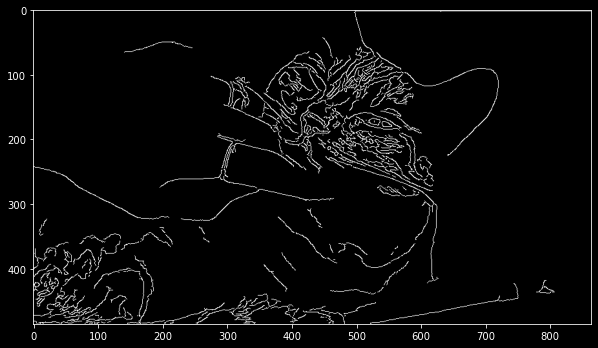## -> 此篇文章的程式碼 github

Day16_高斯模糊找輪廓_GaussianBlur_Canny.ipynb

# 運用 OpenCV 幫助我們找圖片的輪廓(高斯模糊、Canny)

``````shape = (200, 200, 3)
origin_img = np.zeros(shape, np.uint8)
origin_img = draw_cirlces(origin_img, circle_mid=(50,50), color=(0,255,0) ,radius=30)
origin_img = draw_cirlces(origin_img, circle_mid=(50,150), color=(255,255,0) ,radius=27)
origin_img = draw_cirlces(origin_img, circle_mid=(100,100), color=(255,0,255) ,radius=23)
origin_img = draw_cirlces(origin_img, circle_mid=(150,60), color=(255,0,0) ,radius=25)
origin_img = draw_cirlces(origin_img, circle_mid=(150,150), color=(0,0,255) ,radius=21)
origin_img = draw_cirlces(origin_img, circle_mid=(120,35), color=(0,255,255) ,radius=18)
origin_img = draw_cirlces(origin_img, circle_mid=(110,150), color=(255,255,255) ,radius=40)

print("origin picture:")
show_img(origin_img)
``````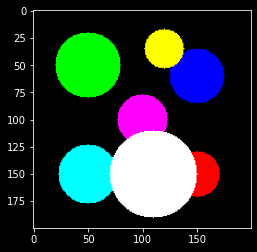## 第一步 - 轉成灰階

``````gray = cv2.cvtColor(img, cv2.COLOR_BGR2GRAY) # start from BGR -> gray
``````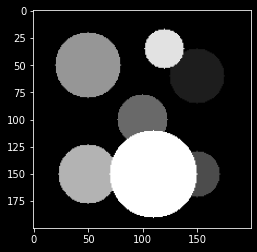## 第二步 - OpenCV 的高斯模糊

``````# 高斯模糊(高斯平滑)，與計算核心大小
def do_GaussianBlur(gray):
kernel_size = 5
blur_gray = cv2.GaussianBlur(gray,(kernel_size, kernel_size), 0)
return blur_gray
``````

`kernel_size` 是我們進行運算時，對於多大範圍的圖片進行運算。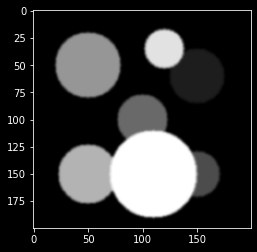(看得出來有比較模糊吧哈哈哈哈哈)

Q: 為什麼要模糊?

A: 因為先做高斯模糊(或說經過高斯平滑)的圖片能夠去除很多圖片的噪聲(雜訊)，

## 第三步 - OpenCV 的 Canny 邊緣檢測

``````# Canny邊緣運算
def do_Canny(blur_gray):
low_threshold = 1
high_threshold = 90
edges = cv2.Canny(blur_gray, low_threshold, high_threshold)
return edges
``````

• 高於 `high_threshold`: 為 `strong edge` ，我們直接保留
• 介於 `low_threshold``high_threshold`: 為 `weak edge`
Canny會檢測 `weak edge` 是否能與 `strong edge` 相連，
如果會相連的才會被保留。
• 低於 `low_threshold`: 我們都不當作 edge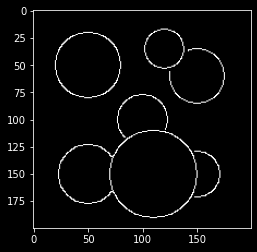# 真實場景運用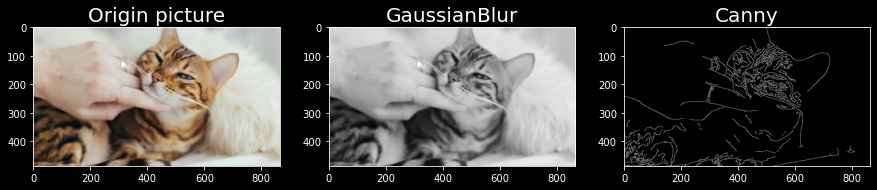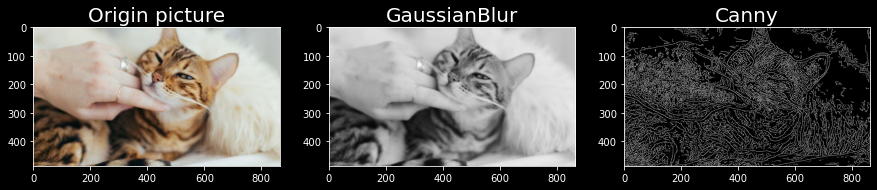(有一種素描畫的感覺?)

`low_threshold, high_threshold`，我們會依據使用情境不同調整參數，